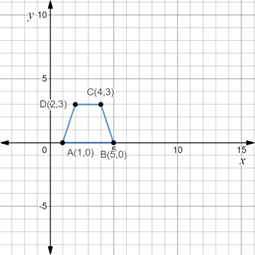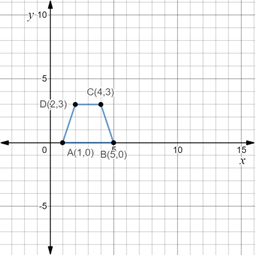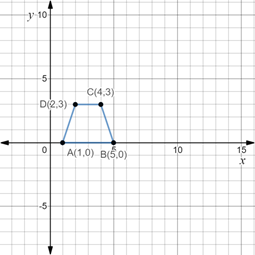# The area and the type of the quadrilateral formed by ABCD along with its figure.### Precalculus: Mathematics for Calcu...

6th Edition
Stewart + 5 others
Publisher: Cengage Learning
ISBN: 9780840068071### Precalculus: Mathematics for Calcu...

6th Edition
Stewart + 5 others
Publisher: Cengage Learning
ISBN: 9780840068071

#### Solutions

Chapter 1.8, Problem 21E
To determine

## To calculate: The area and the type of the quadrilateral formed by ABCD along with its figure.

Expert Solution

The area of the quadrilateral so formed as trapezium is 9 sq. units and its figure is,### Explanation of Solution

Given information:

The four vertices of the quadrilateral A(1,0),B(5,0),C(4,3) and D(2,3) .

Formula used:

Area of a trapezium is half into the product of the sum of its parallel sides and its height. So, if a and b are the parallel sides of the trapezium and h is its height, area of trapezium is calculated as,

A=12(a+b)h

Calculation:

Consider the given four vertices of the quadrilateral A(1,0),B(5,0),C(4,3) and D(2,3) .

Observe that all the points lie in the first quadrant of the coordinate plane, so, plot the points in first quadrant of the coordinate plane and join the vertices to form the quadrilateral as,In the above figure, it is observed that the quadrilateral so formed has one pair of parallel sides, which is the characteristic of the trapezium, hence, the quadrilateral so formed is a trapezium.

Recall that the area of a trapezium is half into the product of the sum of its parallel sides and its height. So, if a and b are the parallel sides of the trapezium and h is its height, area of trapezium is calculated as,

A=12(a+b)h

Here, a=2,b=4 and h=3 , so, area of the trapezium so formed will be calculated as,

A=12(a+b)h=12(2+4)3=1263=9 sq. units

Thus, area of the quadrilateral so formed as trapezium is 9 sq. units and its figure is,### Have a homework question?

Subscribe to bartleby learn! Ask subject matter experts 30 homework questions each month. Plus, you’ll have access to millions of step-by-step textbook answers!